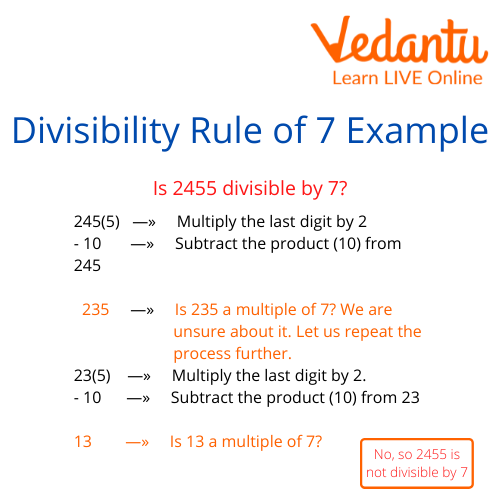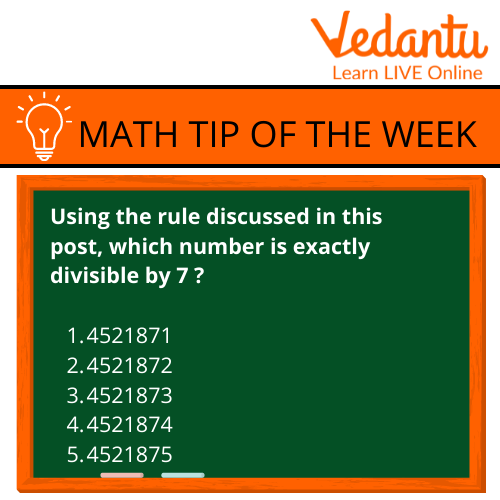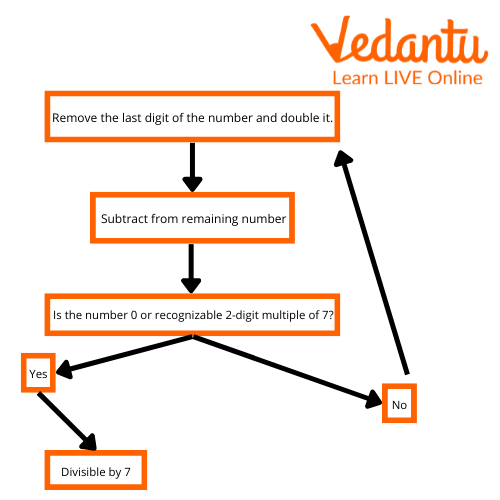Courses
Courses for Kids
Free study material
Free LIVE classes
More

# Divisibility Rule of 7LIVE
Join Vedantu’s FREE Mastercalss

## Introduction to the Divisibility Rule of 7 for Kids

Have you ever heard of the "7" or the "divisibility rule of seven" that many people use today? We will discuss the meaning of the divisibility rule of seven and how it applies to coins and money in this blog post.

Let's begin with the rule of seven's divisibility: Because it divides, the number 7 (7), is referred to as a divisor. When something can be divided into two or more equal parts, it is said to be divisible.Simple Explanation for Divisibility Rule of 7

If one wants to know what a number is divisible by, all one will have to do is divide the individual digits of the number by 7. If any of the digits divide evenly by 7, then the original number is divisible by 7.

## What is the Divisibility Test of 7

Well, first you need to know that there are 7 days in a week, 7 days in a fortnight, and so on. There are 7 colours of the rainbow. A rainbow has 3 primary colours: Red, orange, and yellow.

As a result, there are always seven numbers that may be used to solve any division problem. For example, the number 8 is 1+1+1+1+1+1+1+1, which always divides into the even number 2 as 1+1(2) and the even number 4 as 1+1+1+1(4).

## Where is the Divisibility Rule of 7 used?

The Rule is used in a variety of situations. For example, it can be used when trying to figure out if a number is divisible by 7.

To see how this divisibility test of 7 works, let's look at the numbers 1 to 10. Any integer that is different from the others when divided by seven will have a remainder of 0 or one. Each of these numbers can therefore be divided by 7 without leaving a leftover.Where Divisibility Rule of 7 used

## Which Numbers are Divisible by 7?

7 x 2 = 14 and 14 / 7 = 2.

In the given example, the number divisible by 7 is 14. In this case, the number 14 is divisible by 7 by dividing both digits into 4. (without reducing it to a single digit).

As you can see above the divisibility rule of 7 for example, at each place value of the number (1,2,3, and 4) either of them will divide into a common factor or both of them will separate into an even multiple. This means that all numbers between 1 to 10 are the numbers divisible by 7.

The divisibility rule of 7 is also used in a lot of games over the internet. As we all know that online games, including online casino games, all follow a specific set of rules, which means that no matter what game or slot machine game or table game it is, it will always follow the same rules and it will be fair to everyone. The same goes for cryptologic casino games.

In our opinion, it is one of the ways to learn Maths quickly, because you can use this rule over and over again. At first, you do not understand what it truly means, but as you keep applying it in real-life situations and play games, you begin to grasp the meaning of how many even numbers there are in a set of numbers. After knowing how to check divisibility by 7 this stuff for sure helps to raise your IQ scores!

## Solved Examples

Example 1. Is 154 divisible by 7?

Ans: The last digit in the given number 15 4 (unit digit ) is 4.

We now use the given number without the last digit which is 15.

Subtract twice the last digit 4 from 15:

15 - 2 (4) = 15 - 8 = 7

The result 7 is a multiple of 7 and therefore 154 is divisible by 7.

Checking using long division: 154 ÷ 7 = 22 with remainder 0.

Example 2. Consider the number; 308. check if it is divisible by 7.

Ans: Following the rule:

Double of the last digit =16

Subtracting the result from the rest of the number; 30-16 =14

14 is a multiple of 7, hence the number is divisible by 7.

## Practice QuestionsSums to Practice

Ans: Only (4) 4521874 is divisible by 7

## Summary

We learned how to check divisibility by 7 as well as the divisibility rule of 7 is a fundamental rule that all numbers can be divided by 7 without having any remainder after the division and this is because whenever we divide a number by 7, the resulting number is always even.Divisibility Rule of 7

Last updated date: 20th Sep 2023
Total views: 118.2k
Views today: 3.18k

## FAQs on Divisibility Rule of 7

1. How does the rule of seven work?

This rule works on the basis that if you take individual cell numbers from a number, and then divide each one into seven, you'll get an even number every time. When something can be divided into two or more equal parts, it is said to be divisible. As you can see from the divisibility rule of seven, for instance, the number will split into a common factor or separate into two even multiples at each place value.

2. How do I apply the rule of seven to my everyday life?

Simply divide each digit of the integer by seven to get how many digits the number can be divided by. The original number is divisible by seven if any of the digits divide evenly by seven. It is primarily used in mathematics and numerology showing the divisibility rule of seven for example. But it can be applied elsewhere as well – for instance, when dividing a cake between two people or assigning seating arrangements at a wedding or party.

3. Is a divisor a factor?

The divisor is any number that divides another number. A factor, however, is a divisor that divides the number entirely and leaves no remainder. So, all factors of a number are its divisors. But not all divisors will be factors.